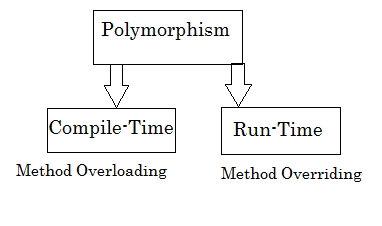Monday, September 24, 2018

Understanding Object Oriented Concepts - Polymorphism

Polymorphism is the core feature of Object Oriented Programming. Polymorphism means one action can be performed in different ways where Poly means many and morphism means forms.

example : customer can perform one operation to check his balance.

If he is mobile customer then mobile phone balance will shown, if he is bank customer then bank account balance will shown.

That means one operation "checking balance" works differently according to customer.This is the polymorphism.

This features allows that an object can have multiple same name functionalities with their different operations. It serves re-usability in programs.Introduction of Polymorphism. ByTechAchievers.com

Compile Time Polymorphism

When same name functions are associated with the same object is known as compile-time polymorphism. Here compiler gets object's reference to call function compile-time or itself.

Compile-Time polymorphism is implemented by Method Overloading or Operator Overloading feature in programming, where object will have same name methods and each method works differently as per the given arguments. Note: Functions/methods can have same name but passing parameters should be different.

Overloading  feature is also known as Static Binding in polymorphism.

Let’s understand an example to calculate area of rectangle, circle and square without using polymorphism.

class Calculate {

public Integer getRectangleArea(Integer length,Integer width) {

return length*width;

}

public Integer getSquareArea(Integer side) {

return side*side;

}

public double getCircleArea(Double r) {

double pi = 3.14d;

return pi*(r*r);

}

}//End of the class

public class mainClass {

public static void main(String args[]) {

calculate calc = new Calculate();

//passing values of length and width
System.out.println(calc.getRectangleArea(2,4));

//passing values of side
System.out.println(calc.getSquareArea(2));

//passing values of r
System.out.println(calc.getCircleArea(3.5));

}

}//End of main class

Let's see same program using Method overloading. Here we are using same name methods and each methods should have different argument. Return type can be of any type.

class Calculate {

public Integer getArea(Integer length,Integer width) {

return length*width;

}

public Integer getArea(Integer side) {

return side*side;

}

public double getArea(Double r) {

double pi = 3.14d;

return pi*(r*r);

}

}//End of the class

public class mainClass {

public static void main(String args[]) {

calculate calc = new Calculate();

//passing values of length and width
System.out.println(calc.getArea(2,4));

//passing values of side
System.out.println(calc.getArea(2));

//passing values of r
System.out.println(calc.getArea(3.5));

}

}//End of main class

Note: Overloading can be done with constructors and operators as well.

Run-Time Polymorphism

When same name functions are associated with the different objects is known as run-time polymorphism. Here compiler will get object's reference to call function run-time.

Run-Time  polymorphism is implemented by Method Overridingwhere same name functionalities are associated with different objects. Objects should be in parent and child relationship. To know about parent and child relationship in OOPS please go though with Inheritance feature of OOPS.

For example class A has display method and class B has same name method.
class B is derived from class A i.e. A is base/parent class and B is derived/child class.

class A {

void display() {

System.out.println(" Displays A ");

}

}

class B extends A {

void display() {

System.out.println(" Displays B ");

}

}

Here each object has same name method so that compiler will get confuse which method is calling, compiler should have object's correct reference to call correct method. Compiler gets object reference at run-time that is why it is called Run-Time polymorphism.

It also known as Dynamic Binding in polymorphism.Run-Time Polymorphism. ByTechAchievers.com

class Shape {

public void showShapeName() {

System.out.println("Displaying Shape Names");

}

}//End of the class

class Square extends Shape {

public void showShapeName() {

System.out.println("This is Square Shape");

}

}//End of the class

class Circle extends Shape {

public void showShapeName() {

System.out.println("This is Circle Shape");

}

}//End of the class

public class mainClass {

public static void main (String args[]) {

Shape shapeBaseClass = new Shape();

//Base class object
shapeBaseClass.showShapeName();

//child class square object
Square squareSubClass = new Square();

//assign child class reference to base class object
shapeBaseClass = squareSubClass;

shapeBaseClass.showShapeName(); // now square method will call

//child class circle object
Square circleSubClass = new Circle();

//assign child class reference to base class object
shapeBaseClass = circleSubClass;

shapeBaseClass.showShapeName(); // now circle method will call

}

}//End of the class

Thanks for reading, do share and comment your suggestions.